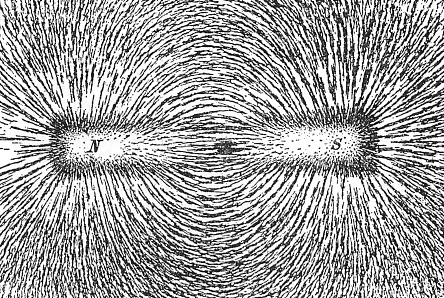# Difference Between Magnetic Field and Magnetic Flux

## Main Difference –Magnetic Field vs. Magnetic Flux

Magnetic field and magnetic flux both refer to properties of magnets. The main difference between magnetic field and magnetic flux is that magnetic field is a region where magnetic poles and moving charges experience a force. Sometimes, the term magnetic field may be also used to refer to the quantity magnetic field strength. Magnetic flux is a measurement of how much magnetic field passes through an area.

## What is a Magnetic Field

A magnetic field is a region in space where magnetic poles and moving charges experience a force (assuming no electric field is present because they can also exert forces on charges). The stronger the magnetic field, the stronger is the force felt. A magnetic field can be depicted using magnetic field lines. A stronger magnetic field has its magnetic field lines drawn closer together. An arrowhead can be drawn on the magnetic field line so that the field lines point along the direction of the path taken by a north pole placed in the magnetic field. The shape of magnetic field lines can be obtained by putting iron filings in a magnetic field and allowing them to line up.If a bar magnet is kept under a piece of paper and iron filings are sprinkled on the paper, then the filings line up along the imaginary field lines.

The magnetic field strength (the magnetic flux density)$\vec{B}$ can be defined using the force$\vec{F}$ felt by a particle of charge$q$ travelling through it at a velocity$\vec{v}$:$\vec{F}=q\vec{v}\times\vec{B}$

If the magnetic field is perpendicular to the direction of particle’s motion, then we have,$F=qvB$

If the term “magnetic field” is used to refer to a quantity rather than a region, it is most likely referring to the magnetic field strength. The SI unit for measuring magnetic field strength is the tesla (T). The Earth’s magnetic field strength changes from place to place, but it is in the order of microteslas. Magnets used in MRI machines in hospitals tend to produce magnetic fields of a few teslas, and the strongest magnetic field we have managed to create is about 90 T.

## What is Magnetic Flux

Magnetic flux is a measurement of how much magnetic field passes through an area. Therefore, this quantity depends not only on the magnetic field strength but also on an area. A simplified explanation for magnetic flux is that the magnetic flux gives the “number” of magnetic field lines passing through the area.

However, the formal definition for magnetic flux is given using vector calculus. In this definition, the magnetic flux$\Phi$ is found by integrating the magnetic field over a surface:$\Phi =\int \vec{B}\cdot d\vec{A}$

For a uniform magnetic field of strength$B$ passing perpendicular to an area$A$, this simplifies down to:$\Phi=BA$

The unit of magnetic flux is, then, T m2. The SI unit for measuring magnetic flux is the weber (Wb), with 1 Wb = 1 T m2.

Gauss’s law of magnetism says that the total magnetic flux through a closed surface is 0. This means that magnetic field lines form closed loops, and therefore, that a north pole cannot exist without a south pole and vice versa. However, there are theories that predict the existence of so-called “magnetic monopoles”, although no experiment has yet discovered them.

## Difference Between Magnetic Field and Magnetic Flux

### What it Describes

Magnetic field describes a region where a magnetic force can be felt.

Magnetic flux describes how much magnetic field passes through an area.

### Dependence on Area

Magnetic field depends only on the magnet that produces it.

Magnetic flux depends not only on the magnet that produces the field but also on the size and orientation of an area.

### Unit of Measurement

The SI unit for measuring magnetic field strength is the tesla (T).

The SI unit for measuring magnetic flux is the weber (Wb); 1 Wb = 1 T m2.

Image Courtesy

“The magnetic field of a bar magnet revealed by iron filings on paper… ” by Newton Henry Black ( Newton Henry Black, Harvey N. Davis (1913) Practical Physics, The MacMillan Co., USA, p. 242, fig. 200) [Public Domain], via Wikimedia Commons